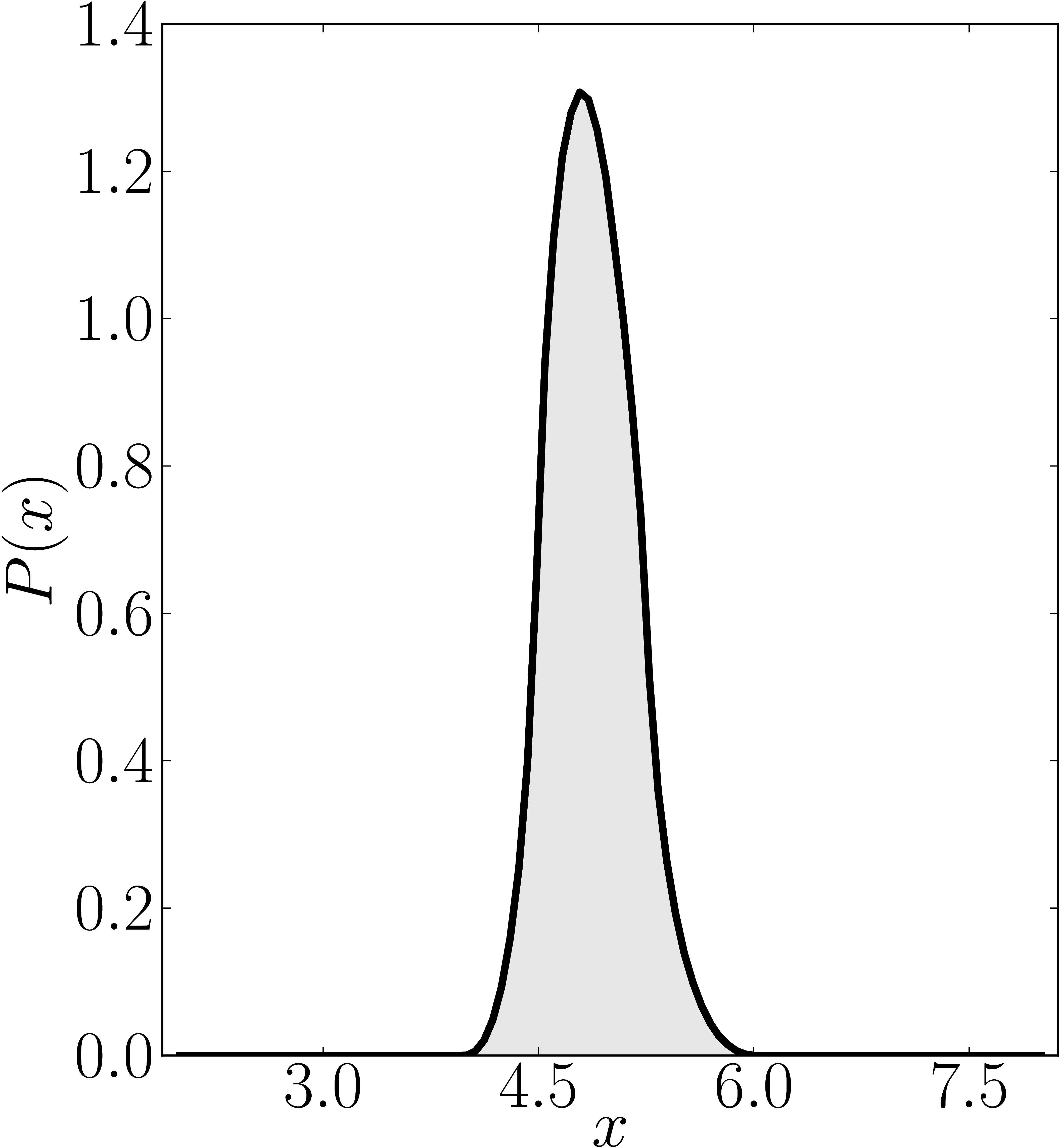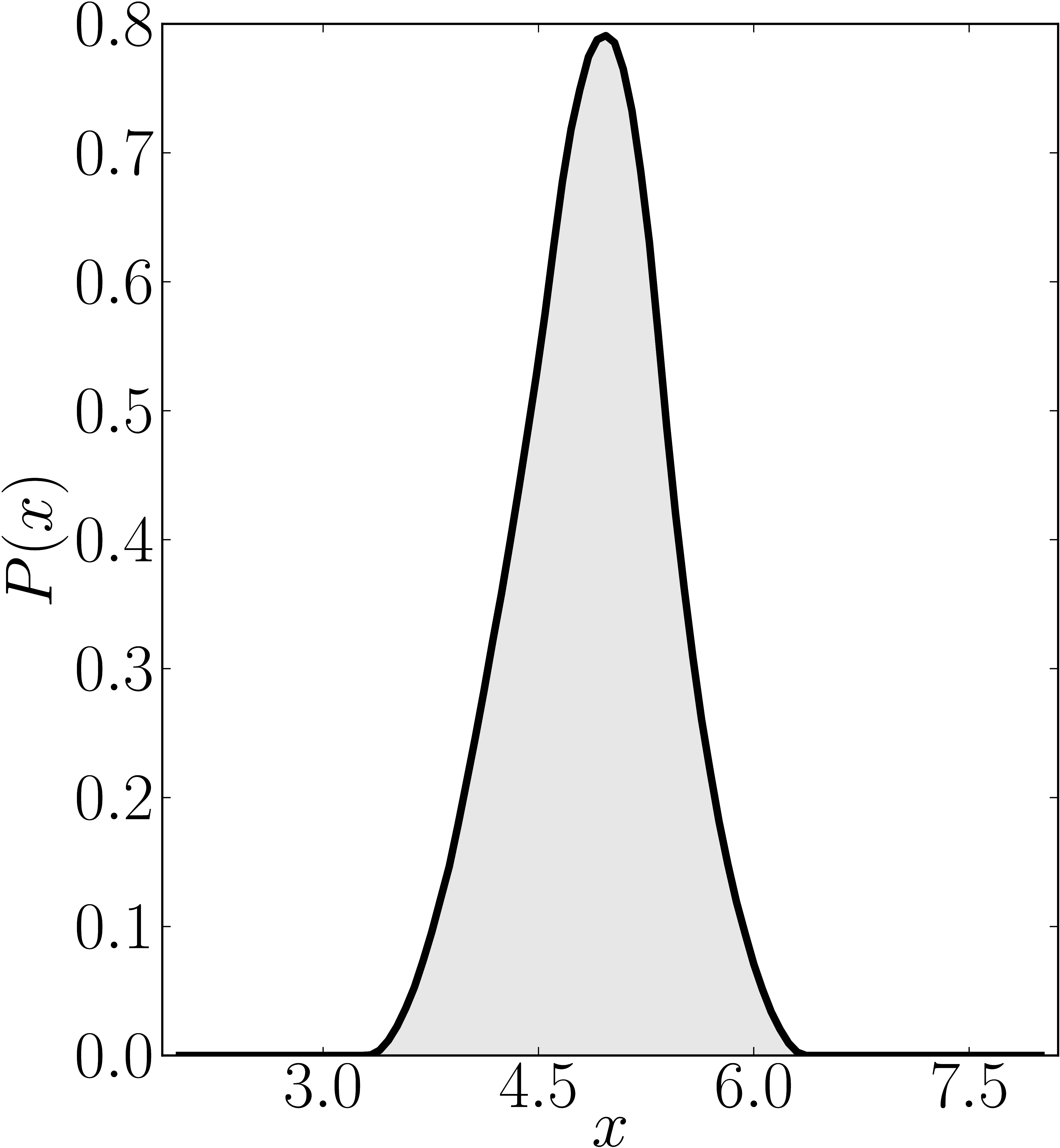# 8x8 matrices

Here we present some example restricted numerical shadow of a 8x8 matrix.

## Diagonal matrices

### Example 1

The matrix is $\mathrm{diag}(1, \ee^{\frac{2 \ii \pi}{3}}, \ee^{\frac{2 \ii \pi}{3}}, \ee^{\frac{-2 \ii \pi}{3}}, \ee^{\frac{2 \ii \pi}{3}}, \ee^{\frac{02 \ii \pi}{3}}, \ee^{\frac{-2 \ii \pi}{3}} 1)$. We present the (restricted) numerical shadow for the following cases:

1. all pure states (standard shadow), separately for complex and real states
2. product states (product shadow), $\ket{\psi}_\mathrm{sep} = U_A \otimes U_B \otimes U_C \ket{000}$
3. GHZ-entangled states, $\ket{\psi}_\mathrm{GHZ} = U_A \otimes U_B \otimes U_C (\ket{000}+ \ket{111})/\sqrt{2}$, separately for complex and real states
4. so-called W-entangled states, $\ket{\psi}_\mathrm{W} = U_A \otimes U_B \otimes U_C (\ket{100}+ \ket{010}+ \ket{001})/\sqrt{3}$.

Here $U_A$, $U_B$, $U_C$ are independent random unitary matrices sampled from $U(2)$ with respect to the Haar measure.

Standard numerical shadow with respect to complex states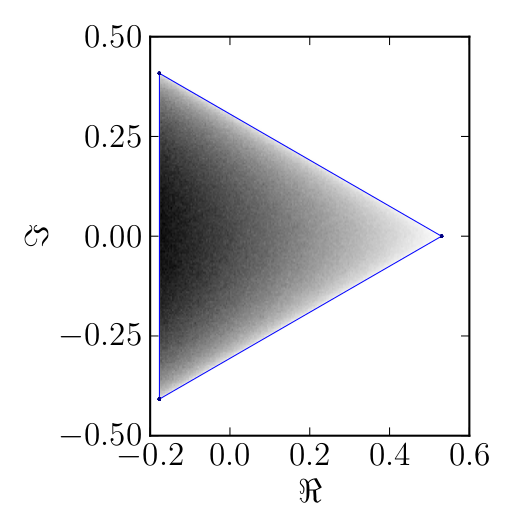Standard numerical shadow with respect to real states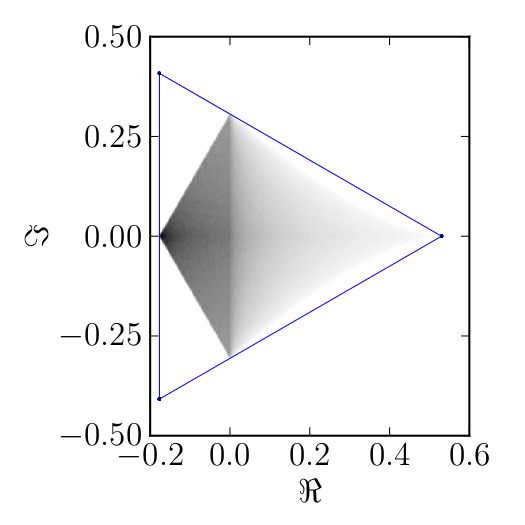Numerical shadow with respect to real separable states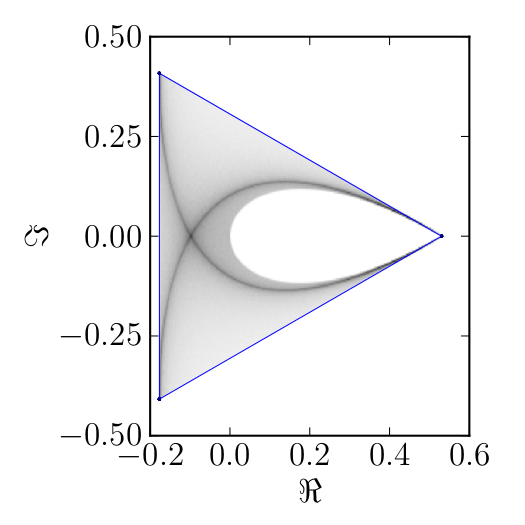Numerical shadow with respect to complex separable states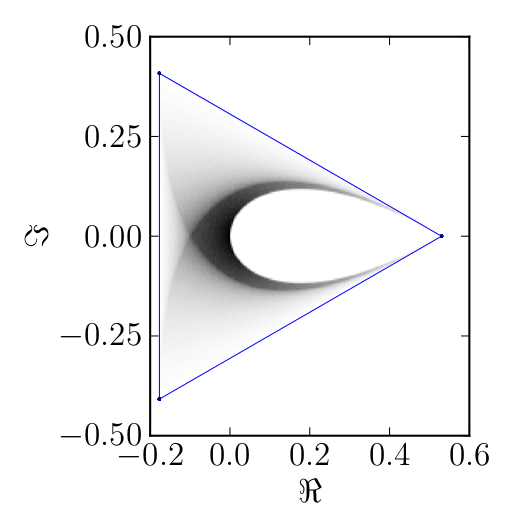Numerical shadow with respect to GHZ-entangled states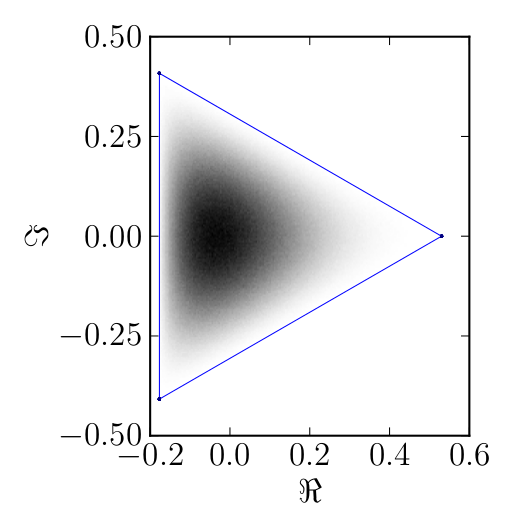Numerical shadow with respect to W-entangled states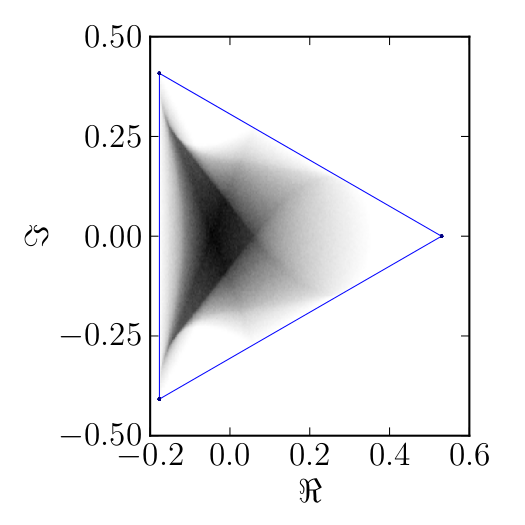### Example 2

The matrix is $\mathrm{diag}(1,2,3,4,5,6,7,8)$

Numerical shadow with respect to complex product $2 \times 2 \times 2$ states. N merical shadow with respect to real product $2 \times 2 \times 2$ states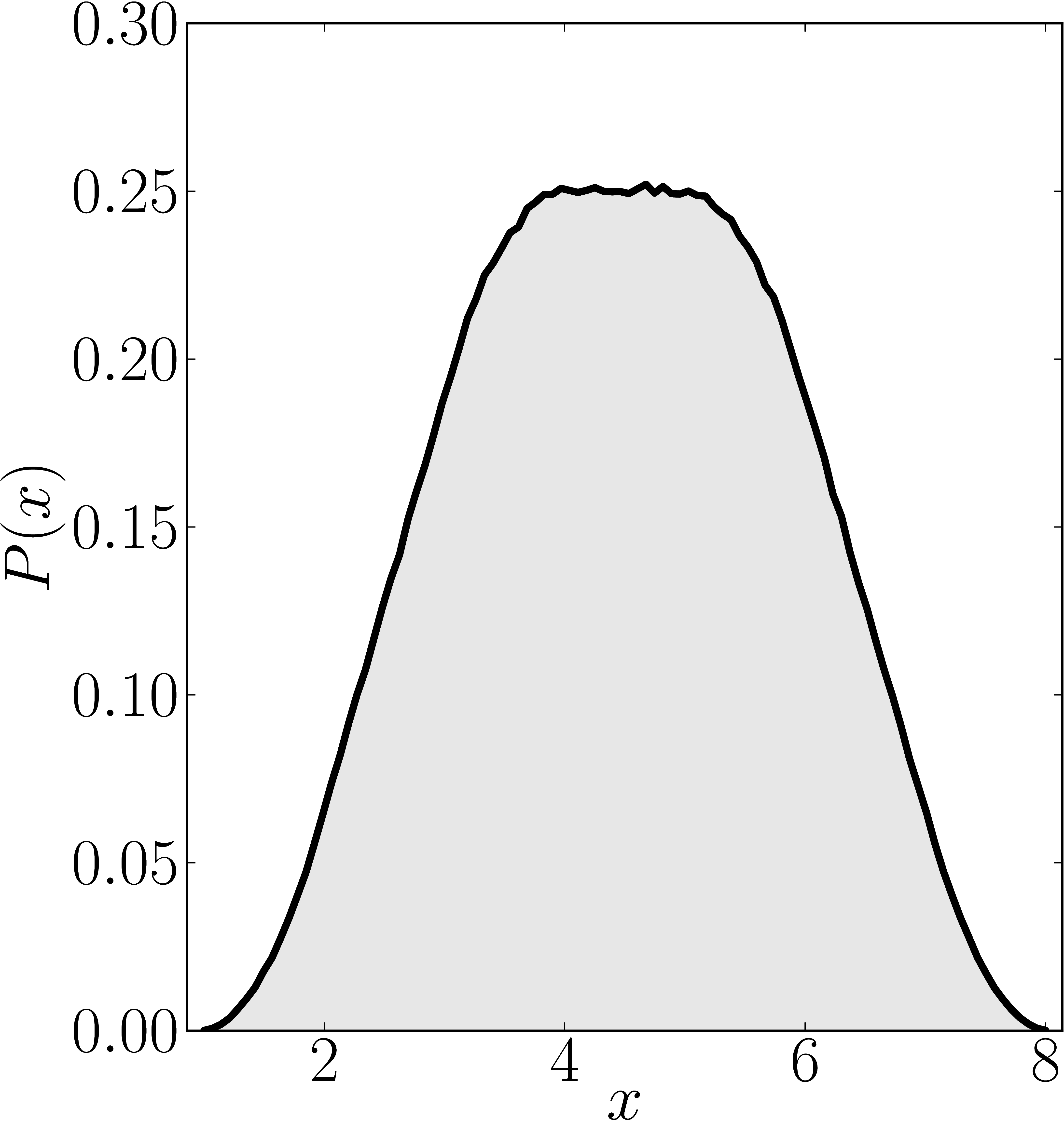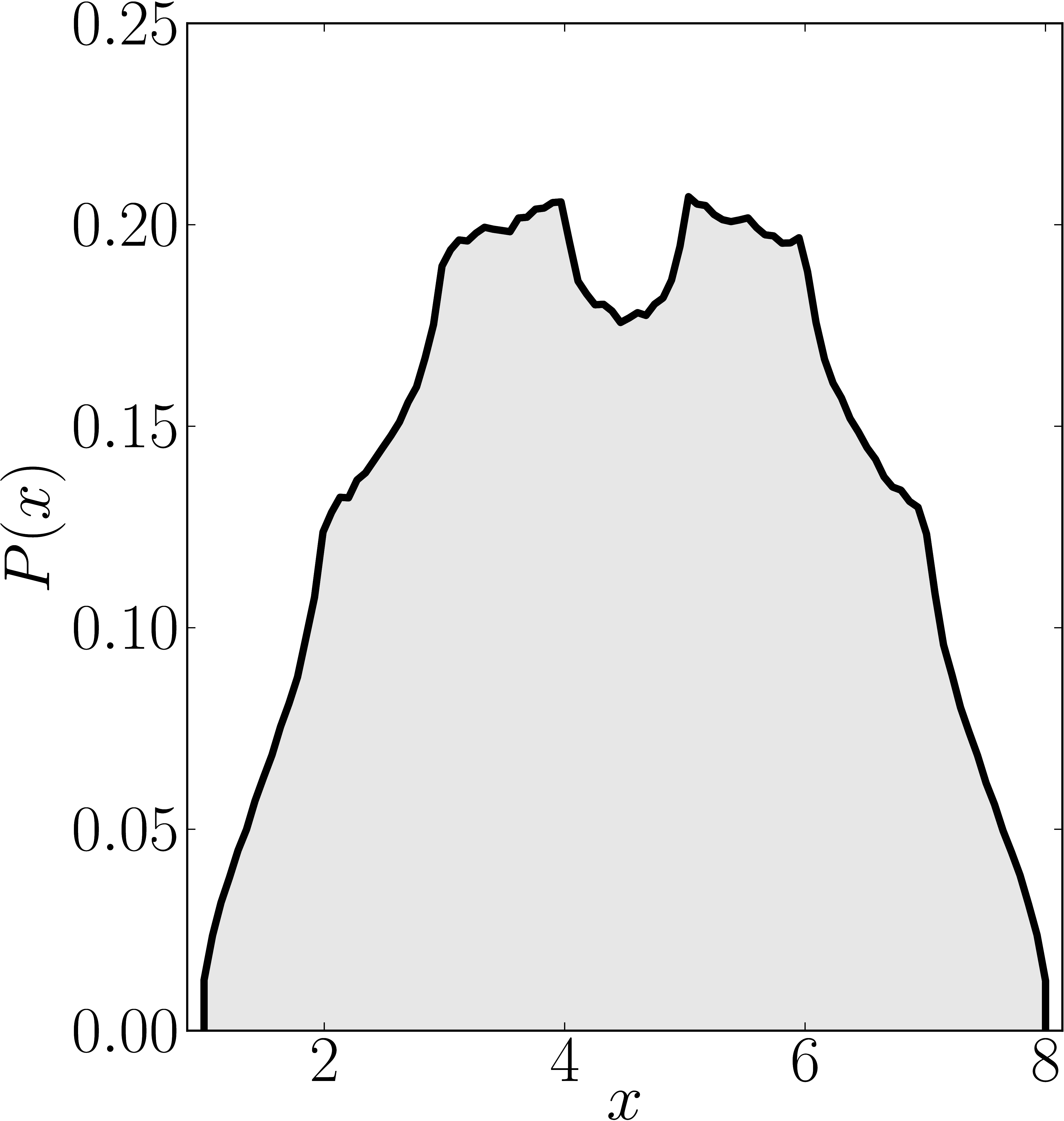Numerical shadow with respect to GHZ states Numerical shadow with respect to W states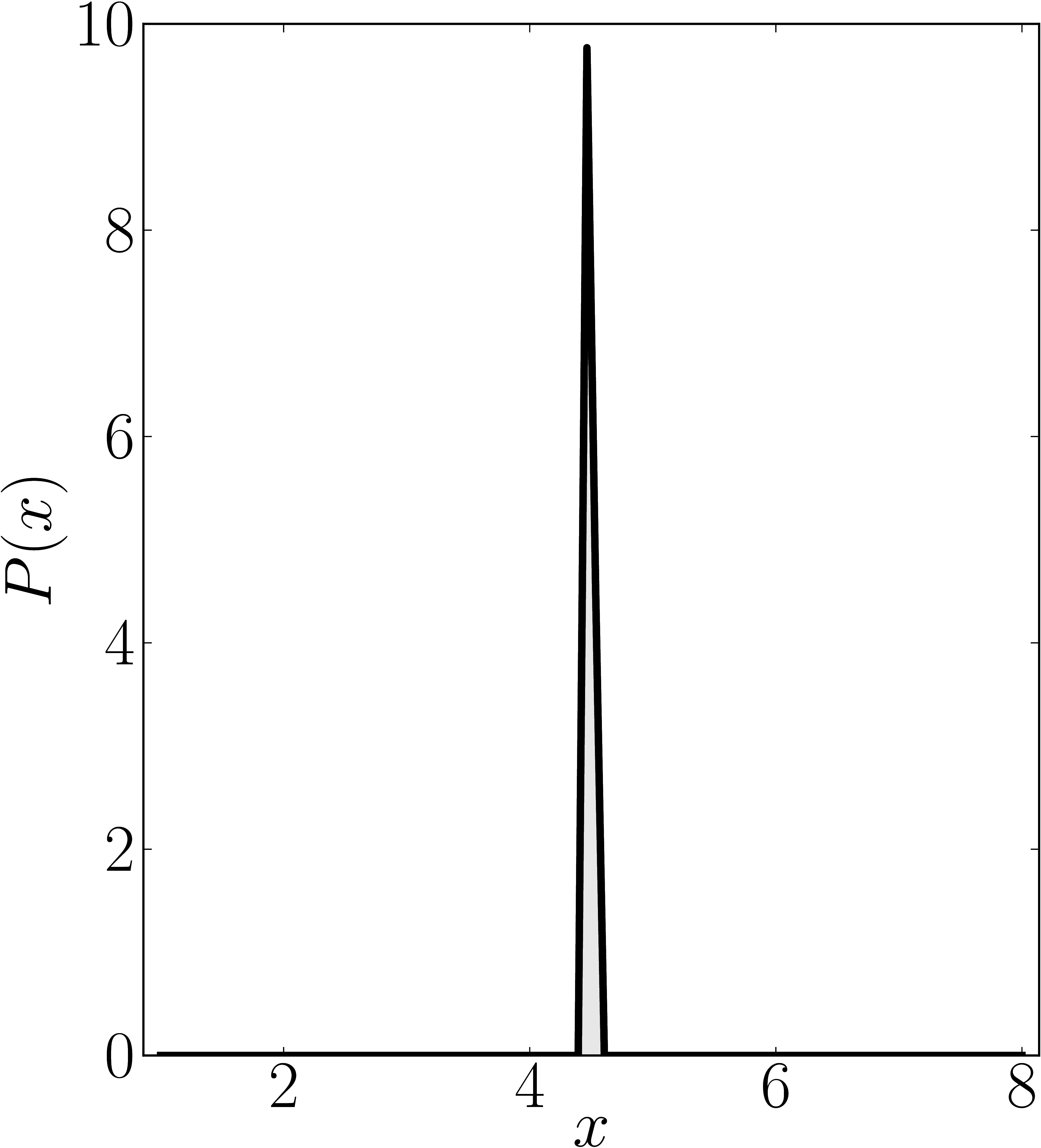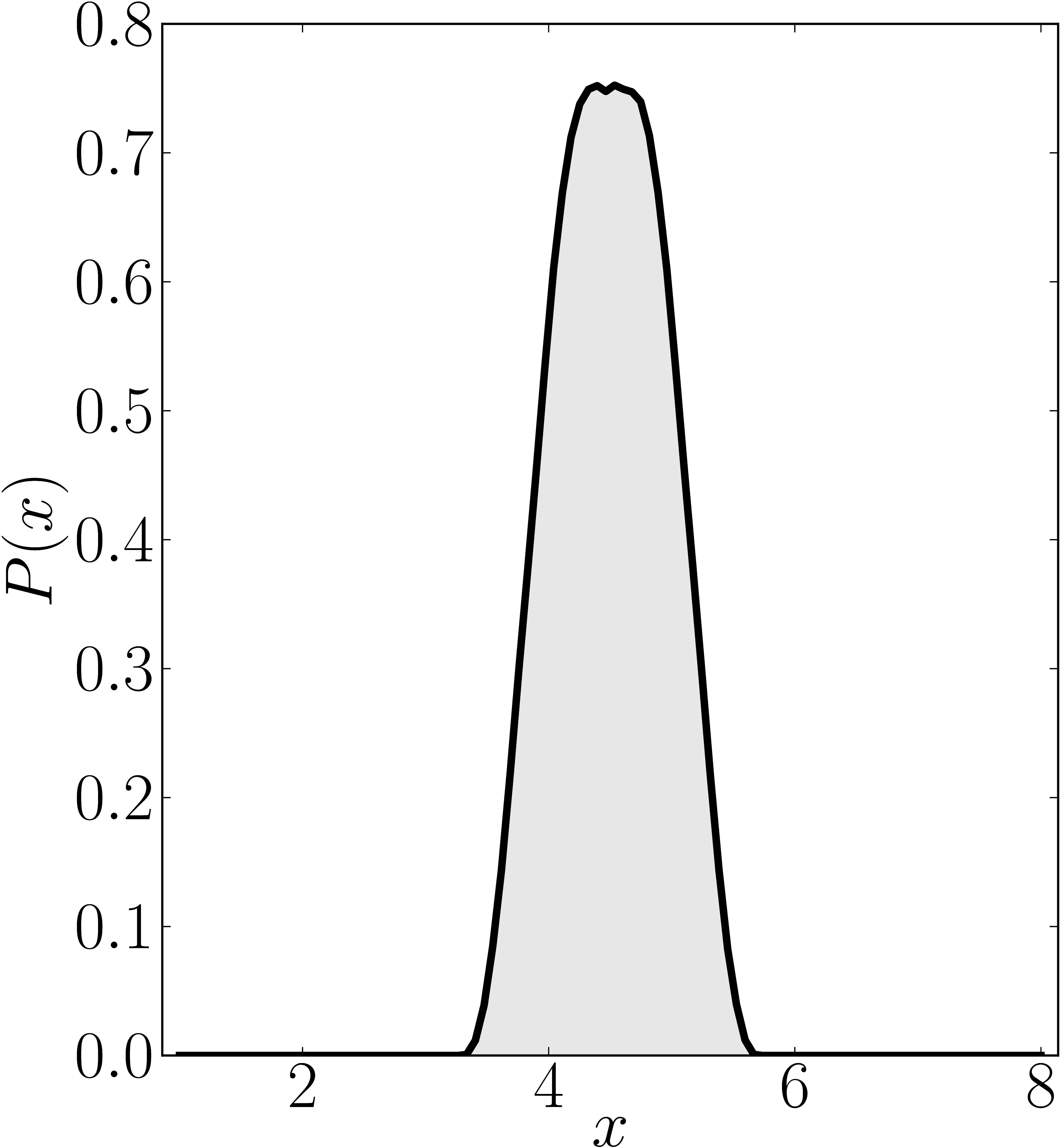### Example 3

The matrix is $\mathrm{diag}(1,2,2,4,5,6,7,8)$

Numerical shadow with respect to complex product $2 \times 2 \times 2$ states. N merical shadow with respect to real product $2 \times 2 \times 2$ states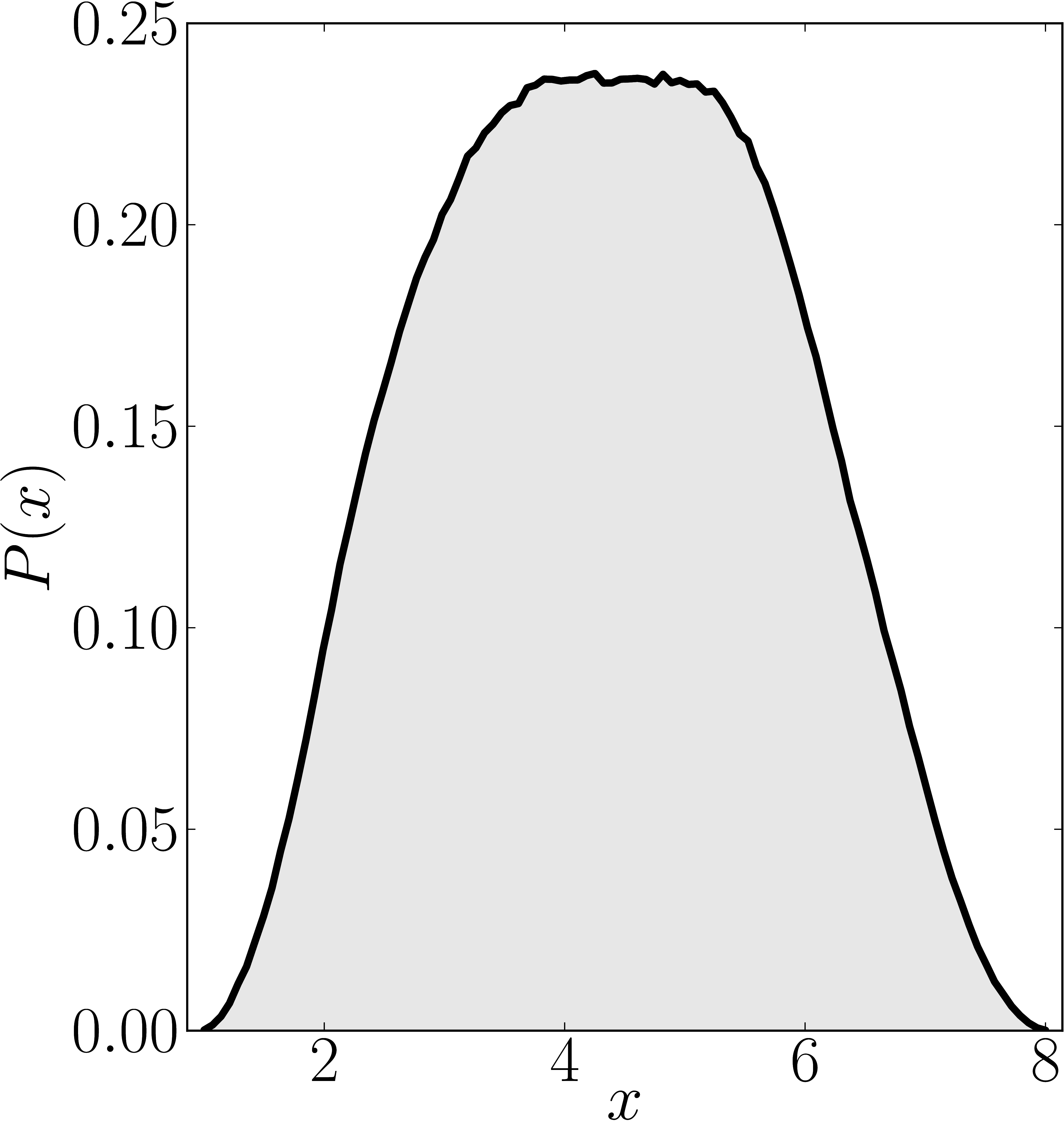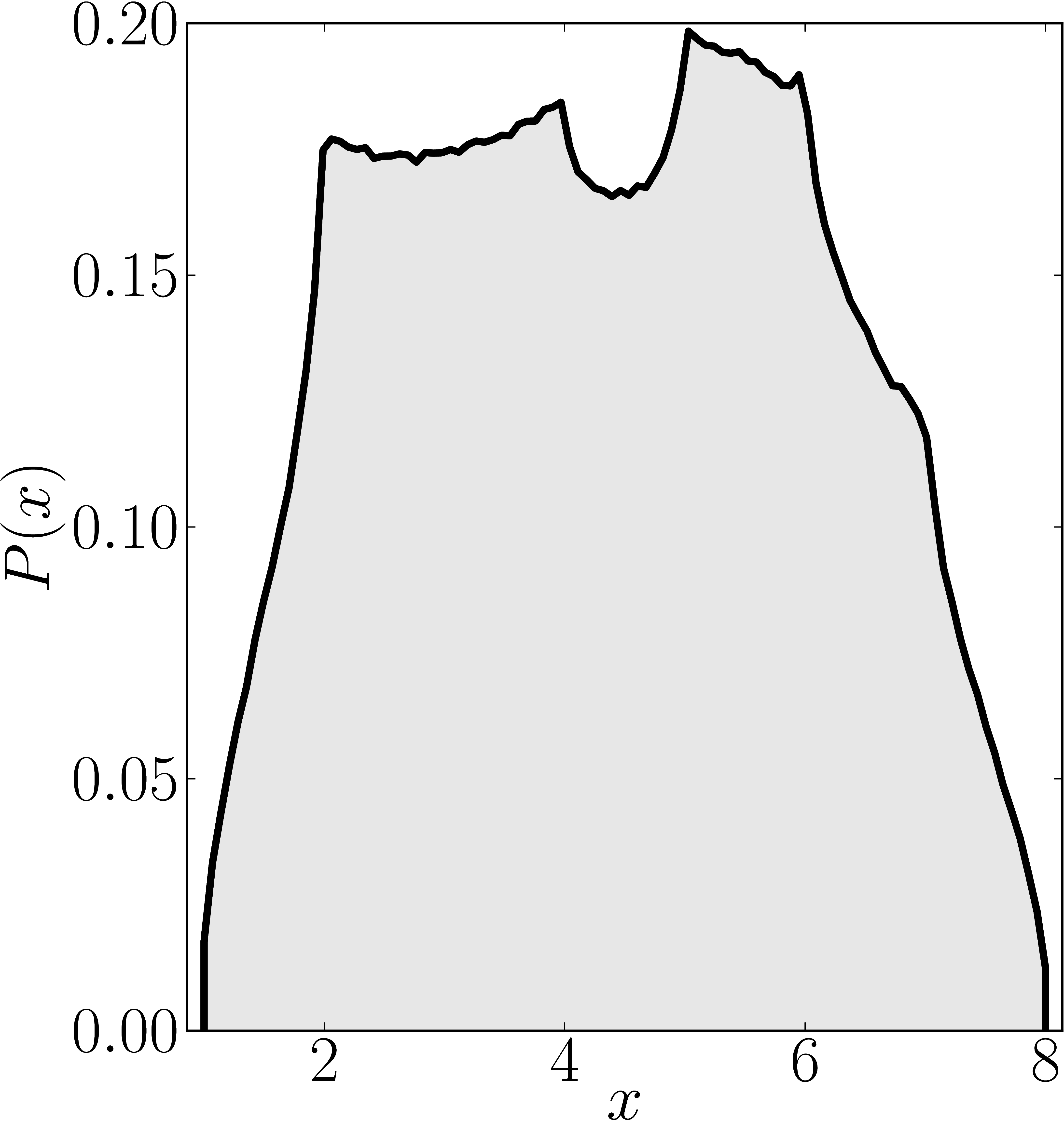Numerical shadow with respect to GHZ states Numerical shadow with respect to W states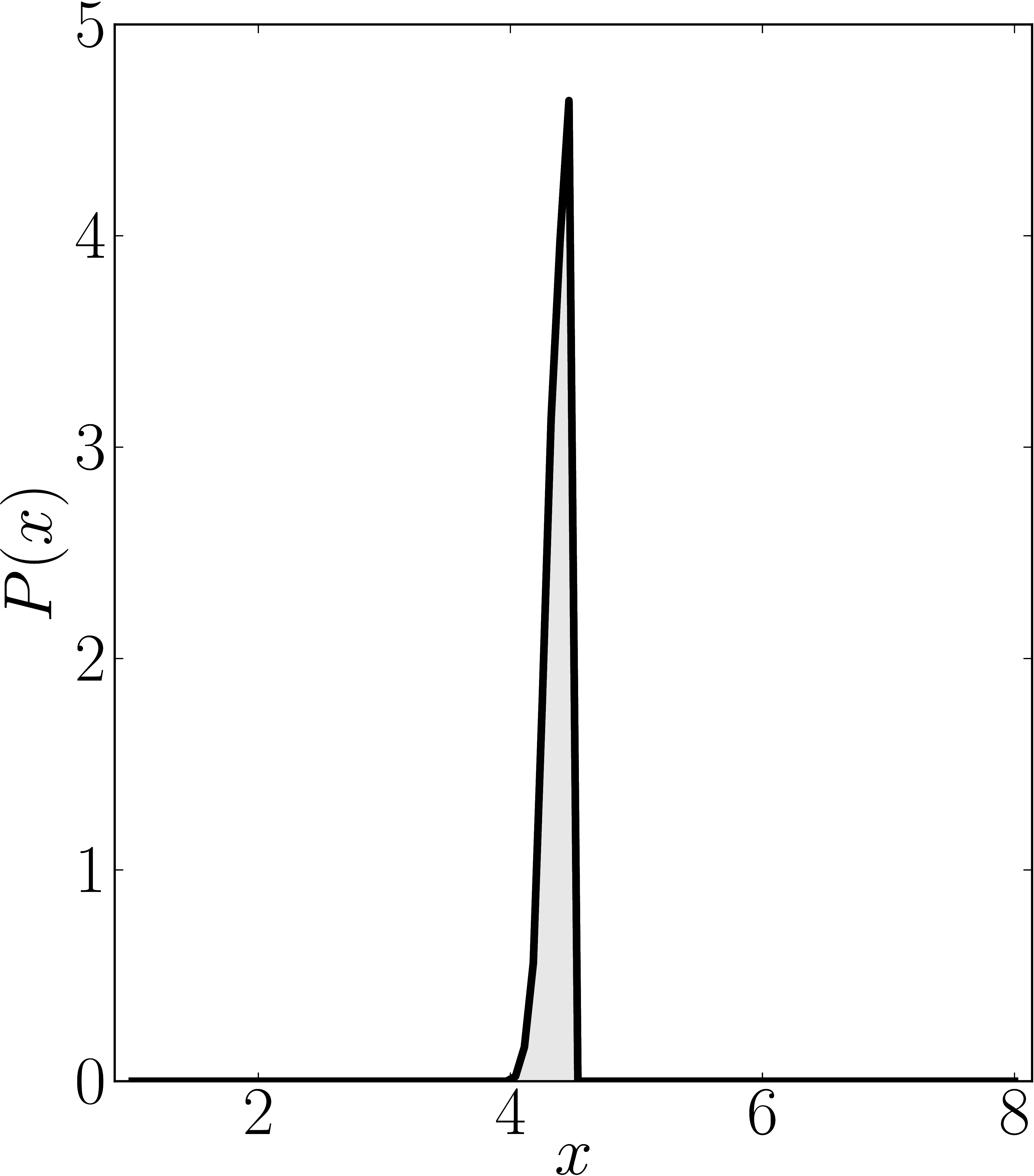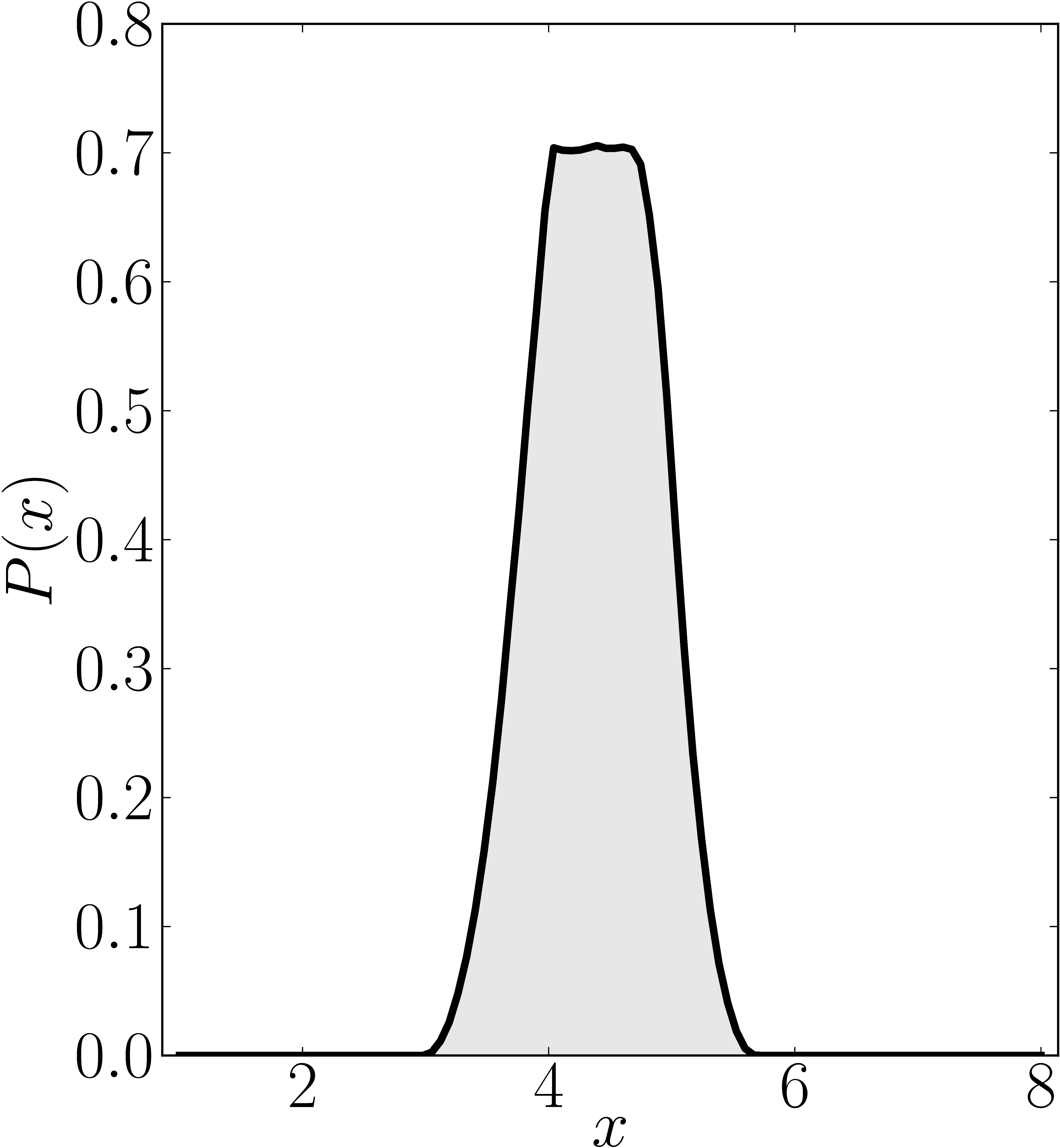### Example 4

The matrix is $\mathrm{diag}(1,2,2,4,4,6,7,8)$

Numerical shadow with respect to complex product $2 \times 2 \times 2$ states. N merical shadow with respect to real product $2 \times 2 \times 2$ states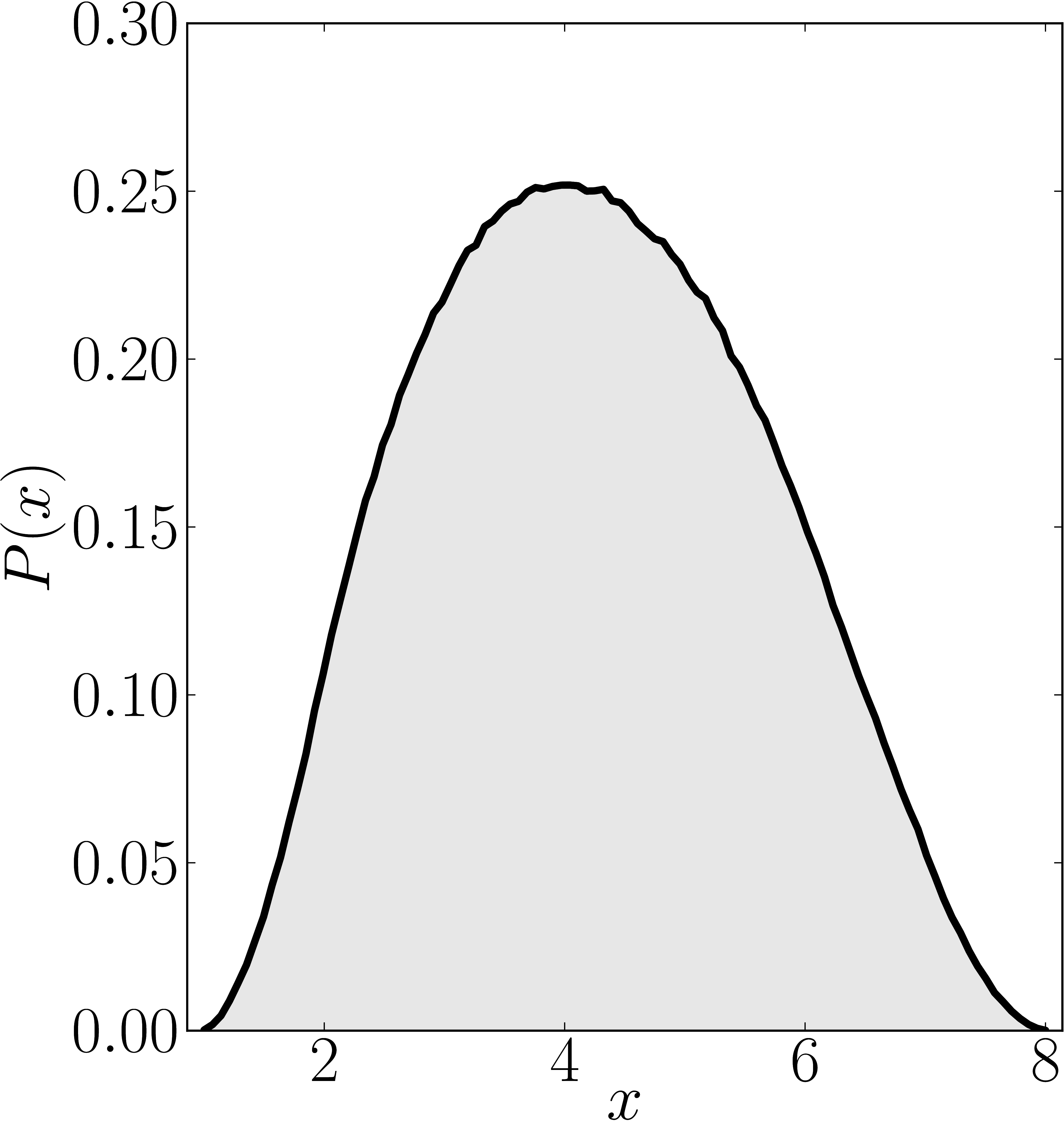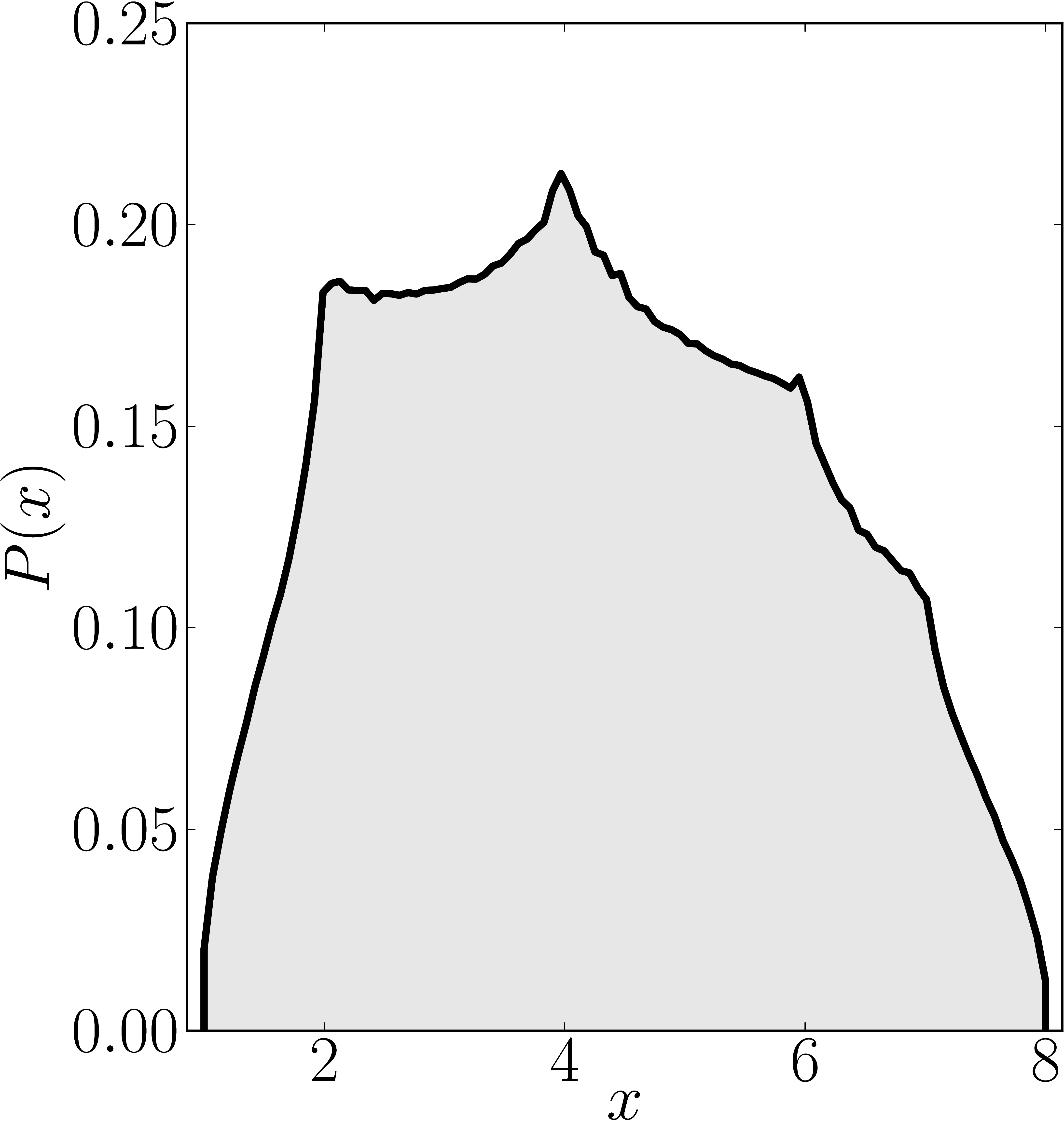Numerical shadow with respect to GHZ states Numerical shadow with respect to W states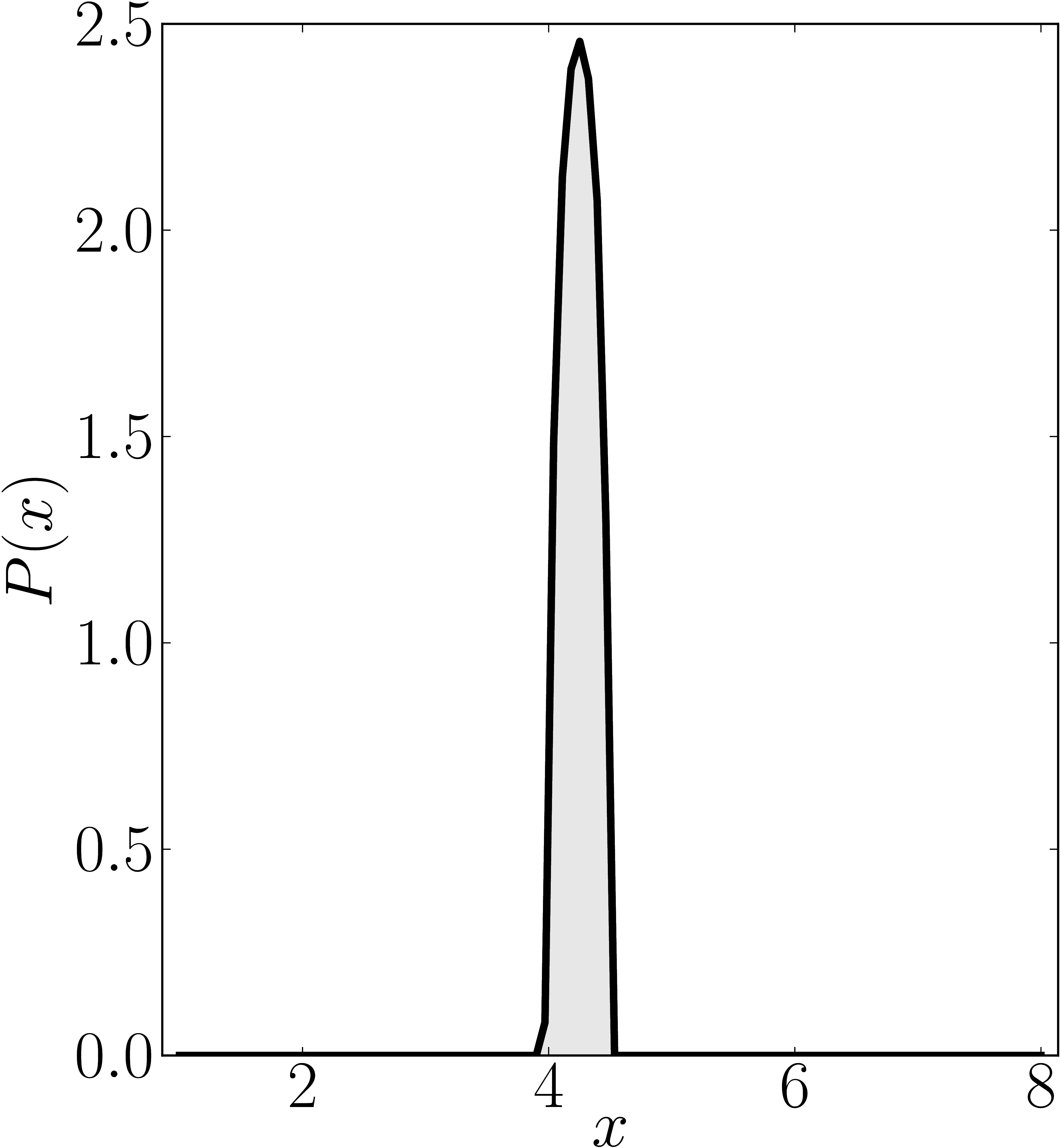### Example 5

The matrix is $\mathrm{diag}(1,2,2,2,5,6,7,8)$

Numerical shadow with respect to complex product $2 \times 2 \times 2$ states. N merical shadow with respect to real product $2 \times 2 \times 2$ states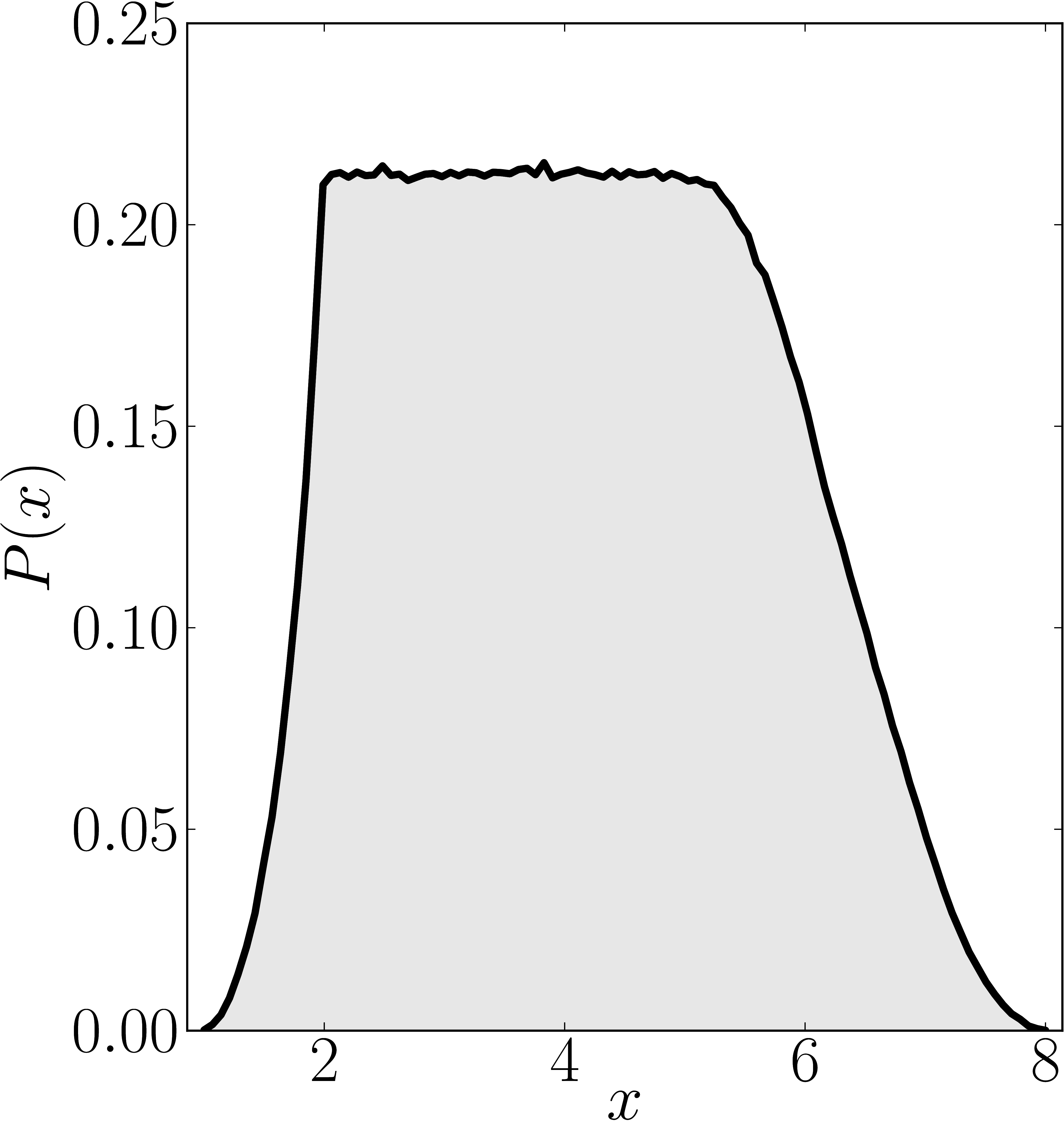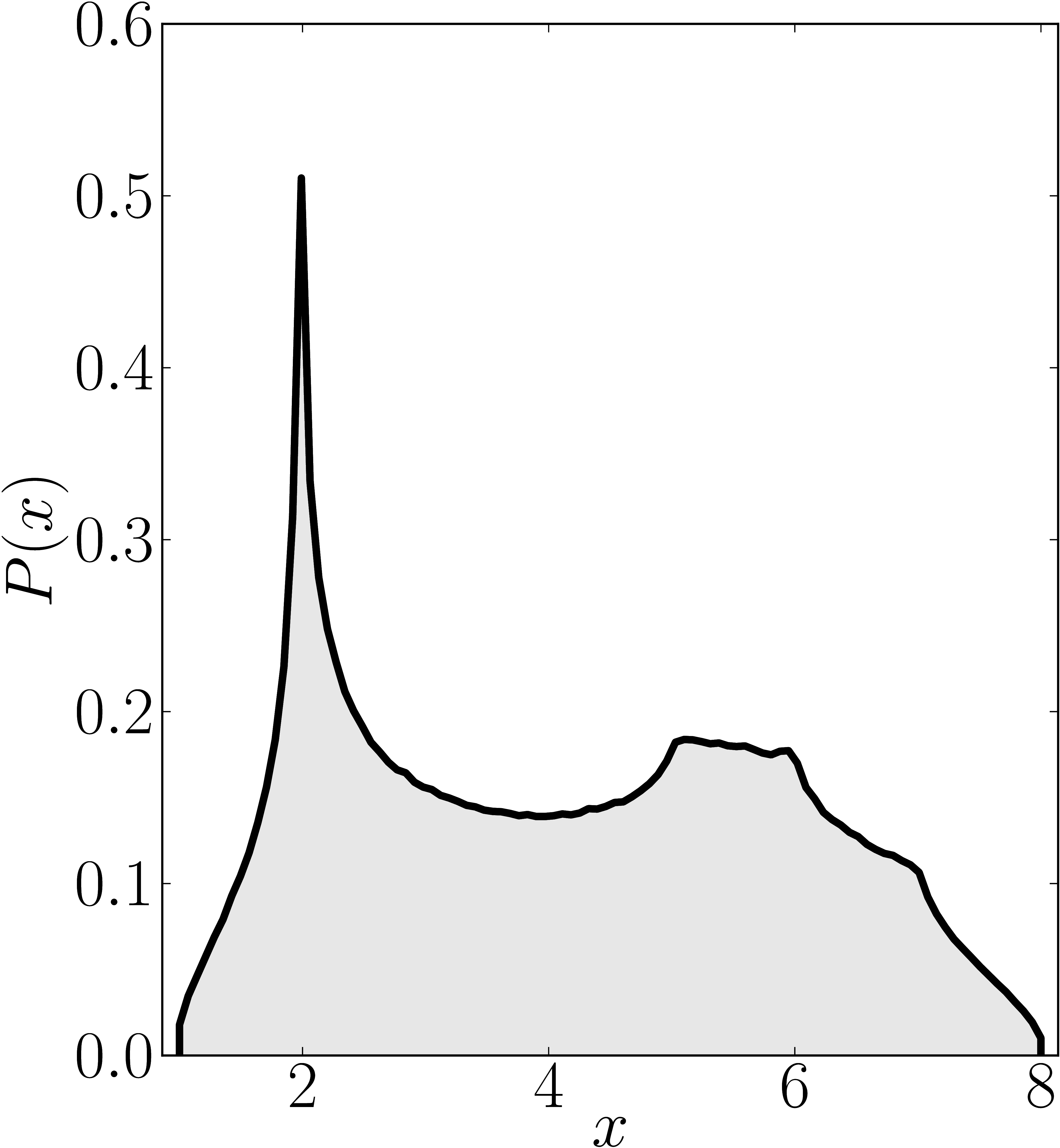Numerical shadow with respect to GHZ states Numerical shadow with respect to W states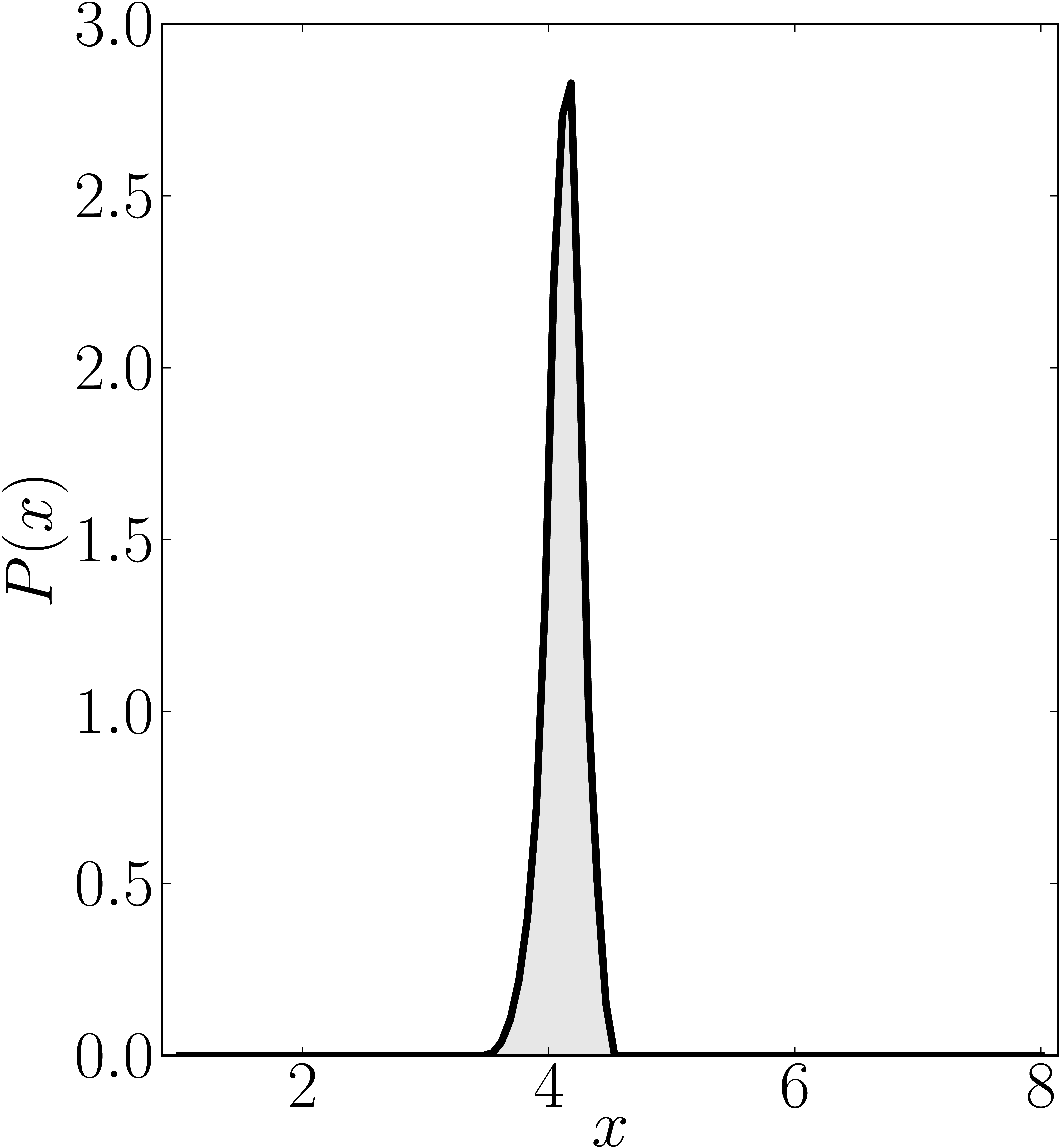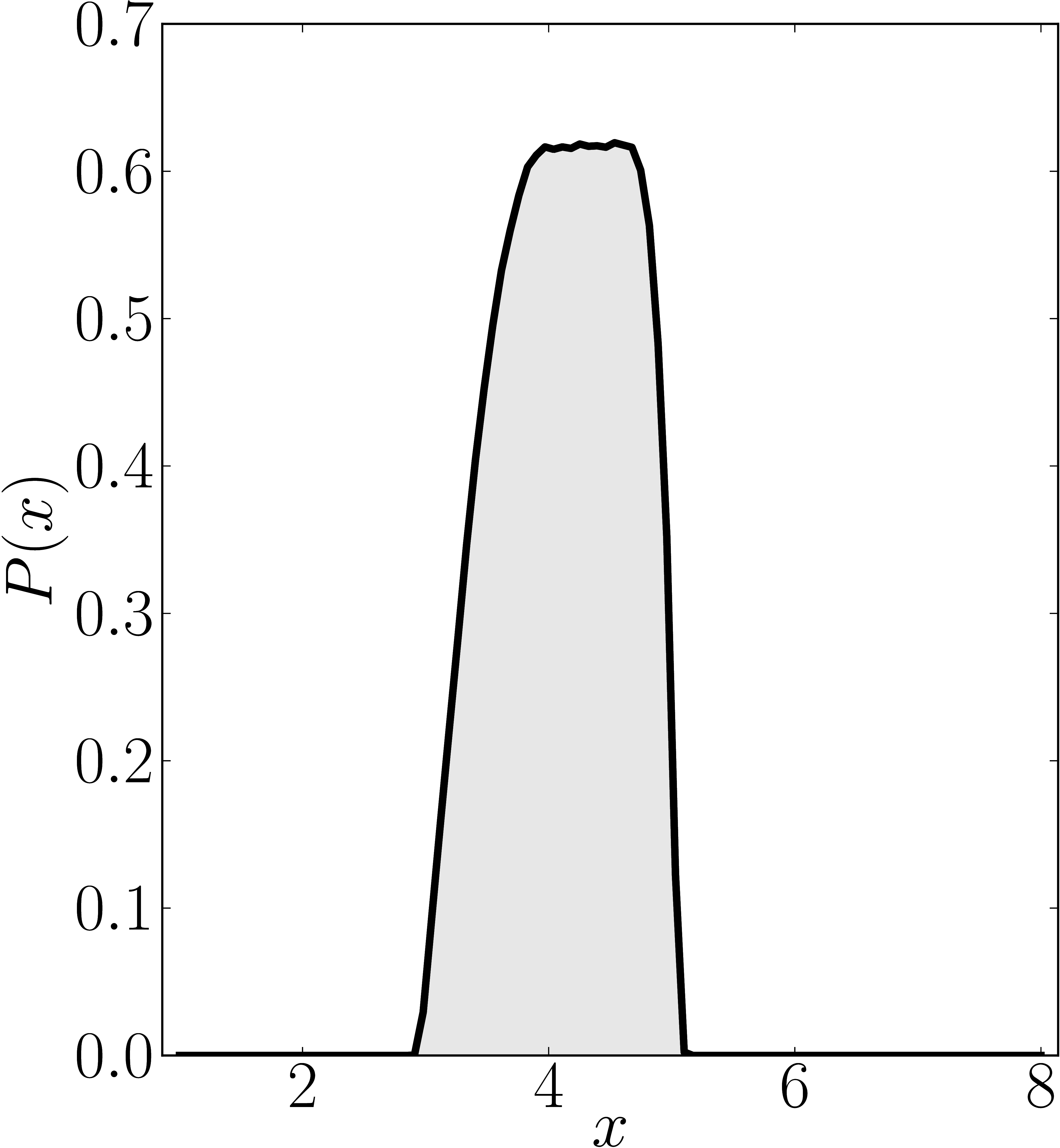### Example 6

The matrix is $\mathrm{diag}(1,2,2,2,2,6,7,8)$

Numerical shadow with respect to complex product $2 \times 2 \times 2$ states. N merical shadow with respect to real product $2 \times 2 \times 2$ states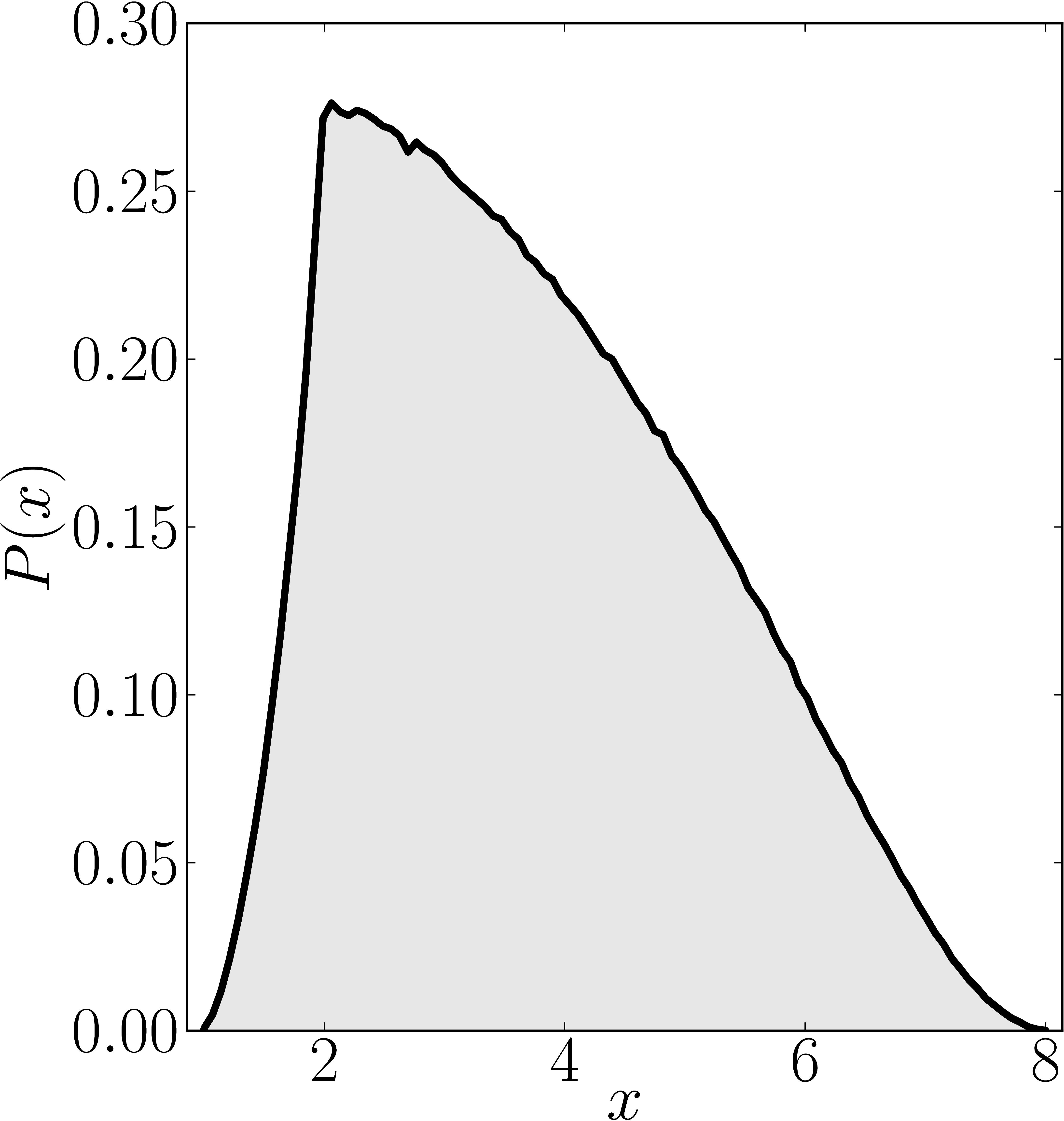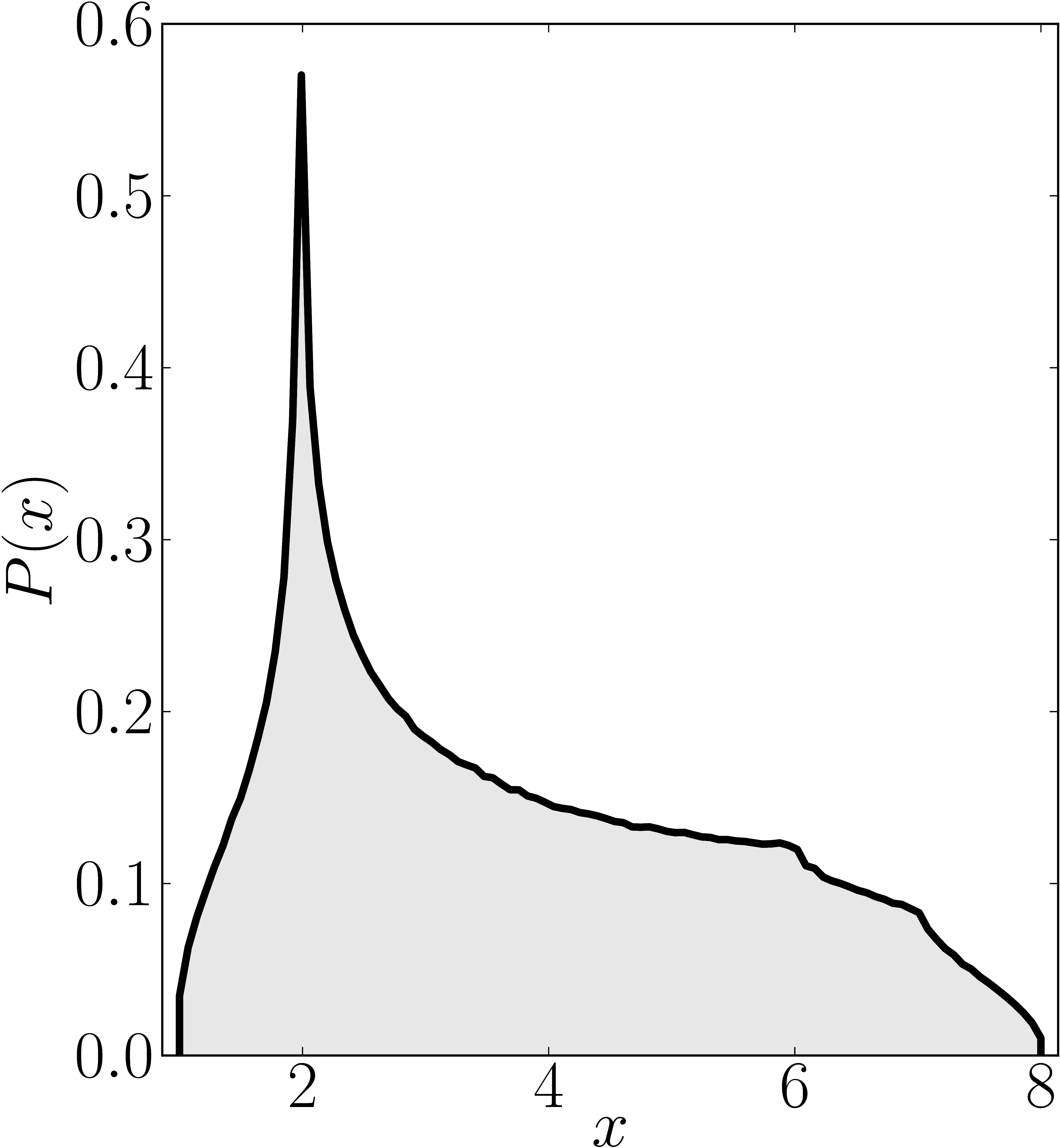Numerical shadow with respect to GHZ states Numerical shadow with respect to W states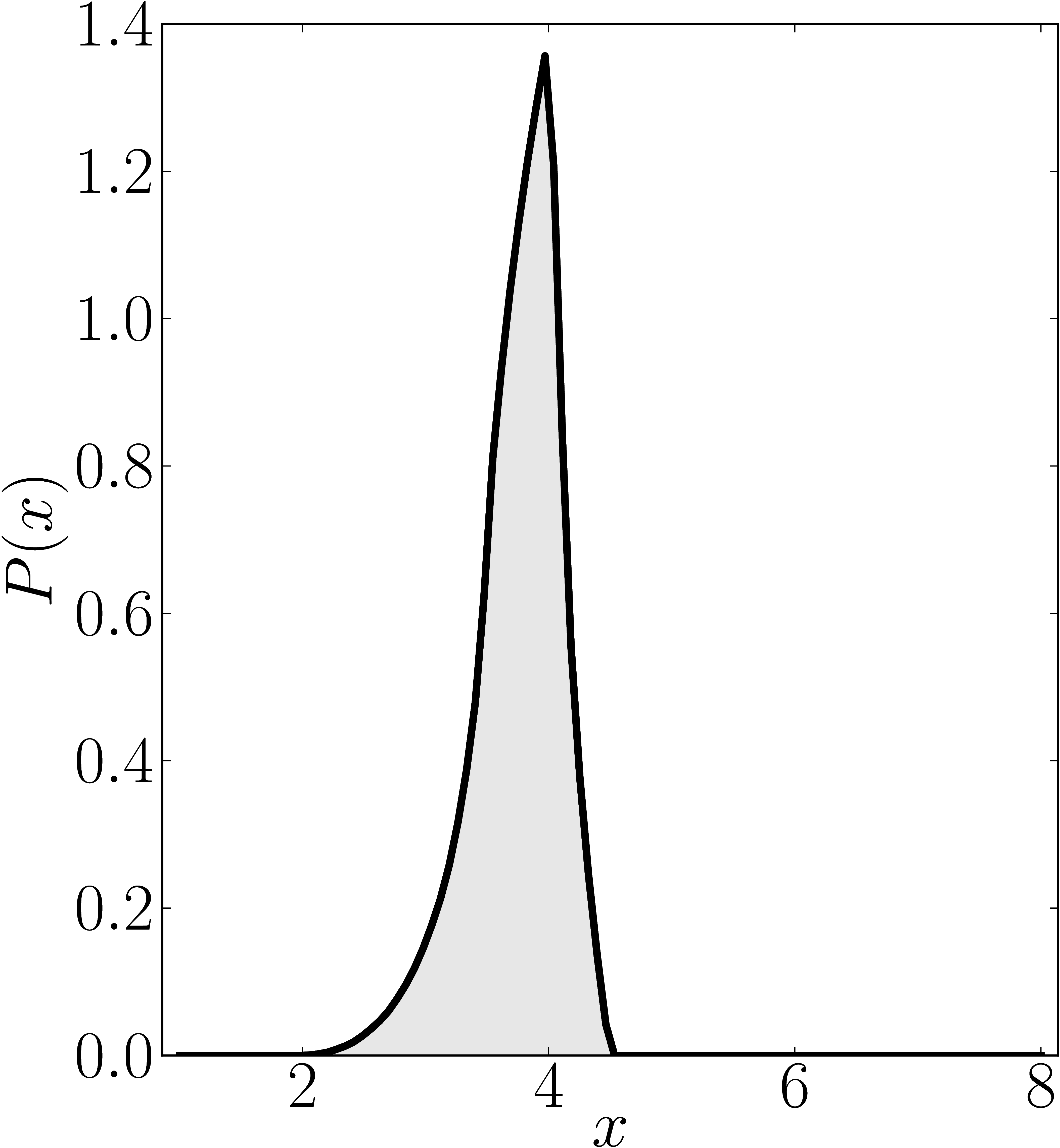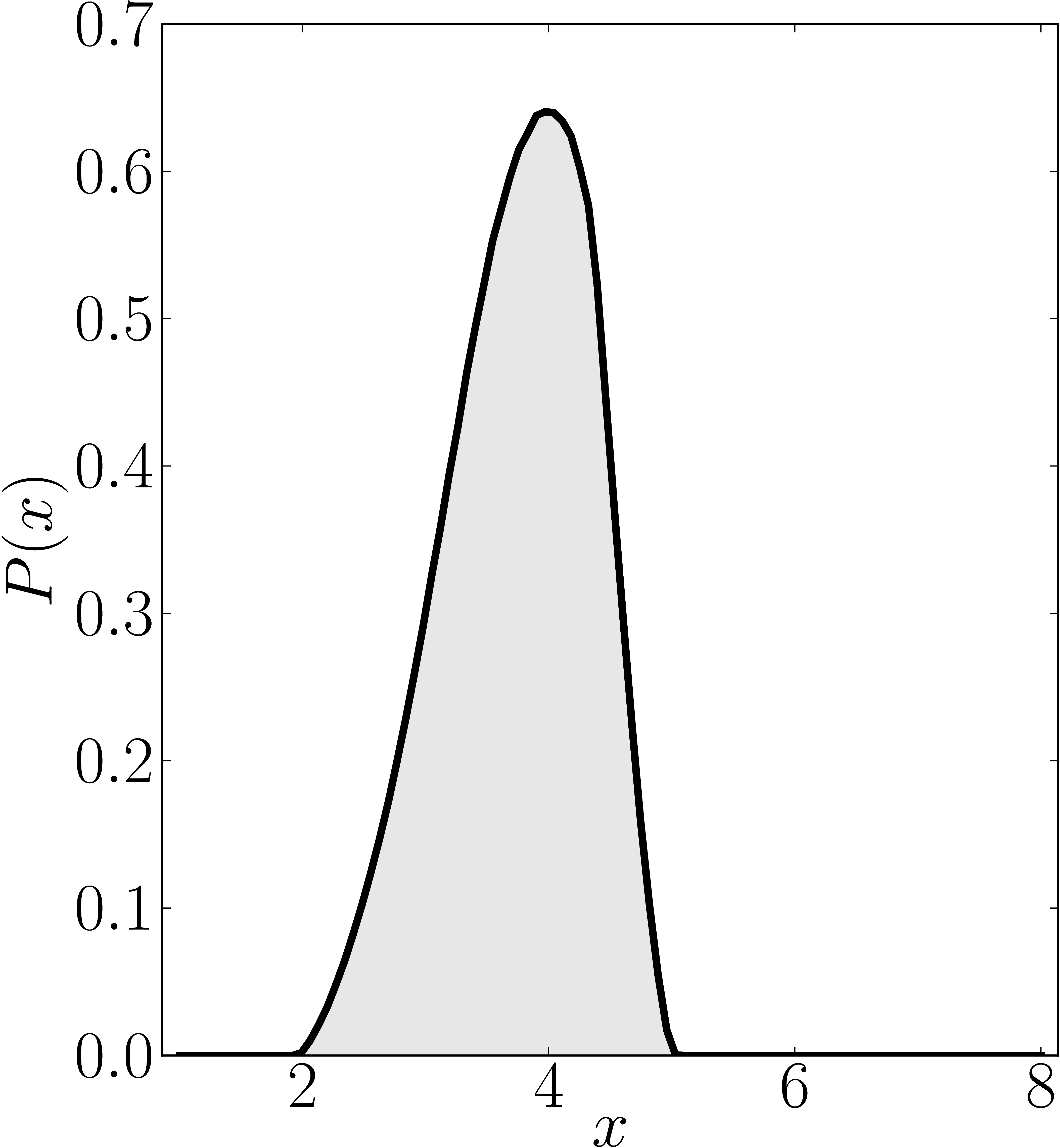### Example 7

The matrix is $\mathrm{diag}(2,2,2,6,6,6,7,8)$

Numerical shadow with respect to complex product $2 \times 2 \times 2$ states. N merical shadow with respect to real product $2 \times 2 \times 2$ states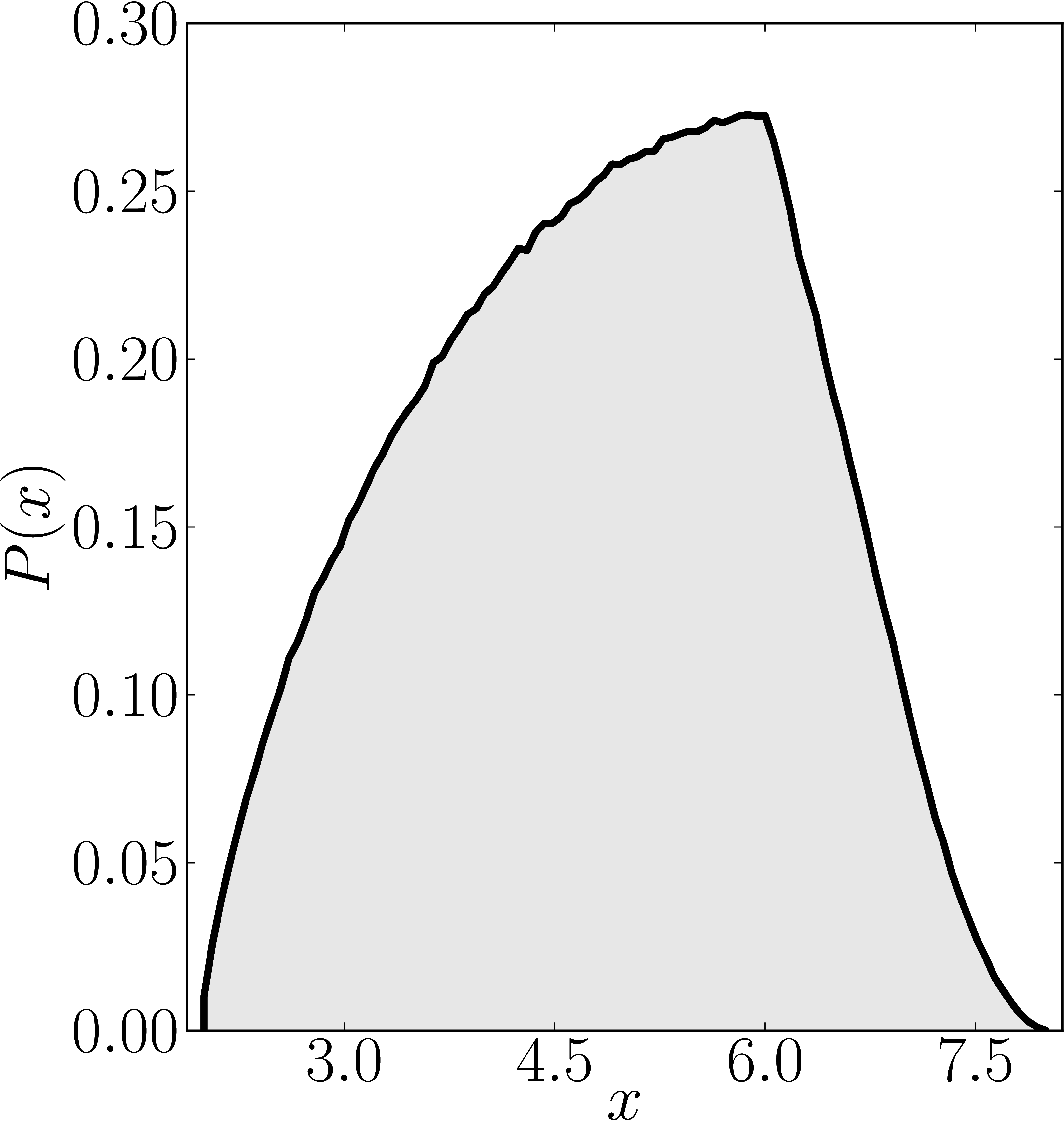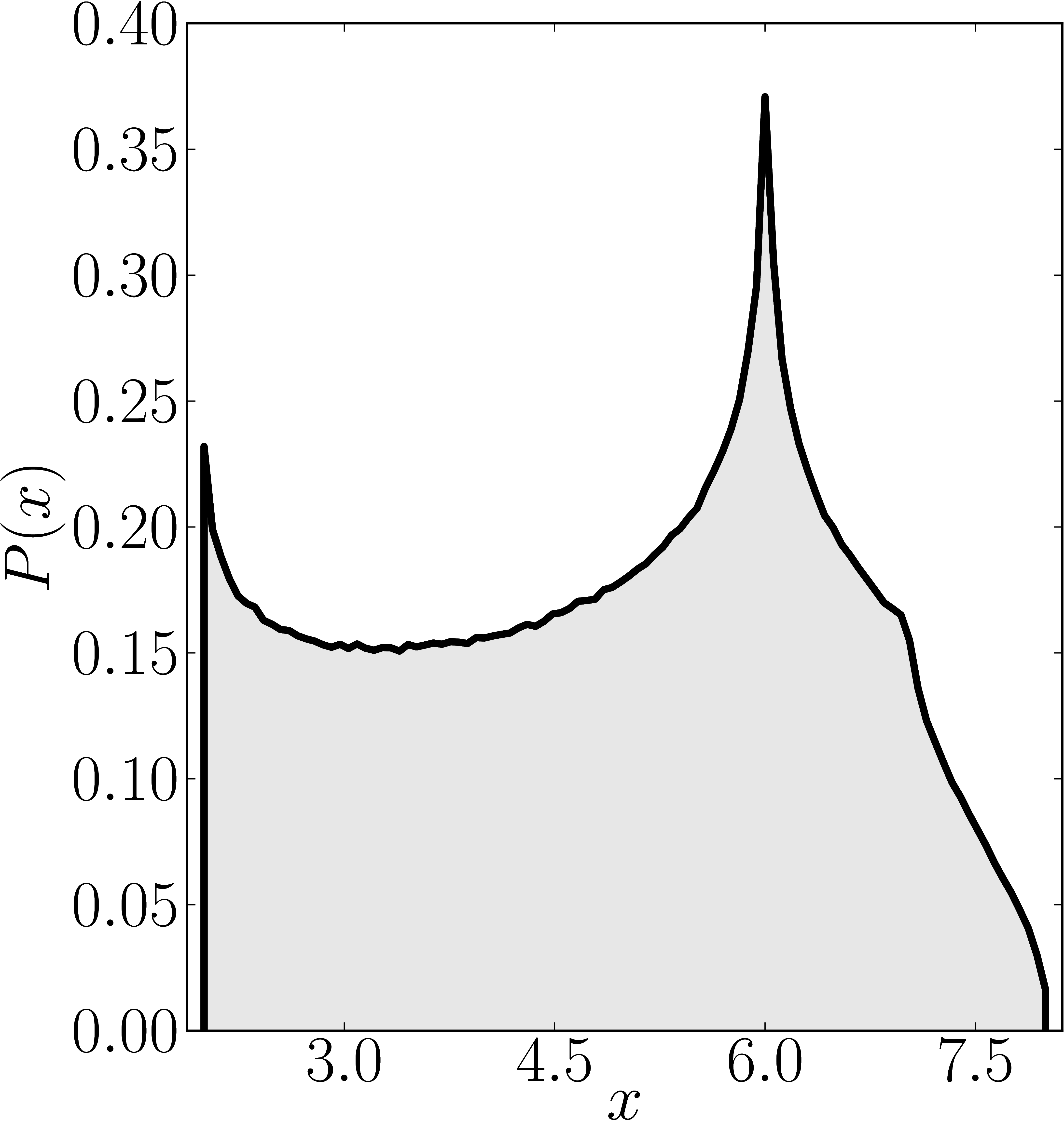Numerical shadow with respect to GHZ states Numerical shadow with respect to W states